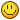## Recommended Posts

Yesterday I had a bit of free time and decided to code a Fibonacci Clock.

The idea is a fresh way of telling the time which is:

• Impractical
• Only accurate to nearest 5 minutes
• Cool af

So... wtf is a fibonacci clock? well... its the idea that every hour interval and 5 minute interval can be coded by a fibonacci value / range.

Fibonacci sequence to those unaware:

1,1,2,3,5...

starting at 1, 1+1 = 2, 2+1 = 3, 3+2 = 5, etc. So Un+1 = Un + Un-1 .

So how is this used as a clock?

Well... you can create any number between 1 - 12 (12 hour clock values) using combinations of numbers from this sequence. For example,

12 Hours = 5 + 3 + 2 + 1 + 1

7 Hours = 5 + 2

2 Hours = 2

3 Hours = 3

11 hours = 5 + 3 + 2 + 1

etc...

As for minutes. every minute value divided by five can be constructed from the sequences

Eg

35 minutes = (5+7) * 5 //the *5 is not coded as part of the clock, this is universal for minutes.

25 minutes = 5 *5

55 minutes = (5 + 3 + 2 + 1 )*5

10 minutes = 2 * 5

Etc.

So. How do u represent this?

Well... the idea is to have a series of boxes, in the golden rectangle dimensions (5x8), split into the corresponding dimensions of each value of the sequence.

So here's what my workspace looked like after a bit of designing:It's all red cause it's midnight.How the colours work:

Red = add to hour only

Green = add to minutes only

(for debugging I added the realtime at the top left. If you do the maths, it's accurate!)

When i say 'add to both', I mean the value of the square (eg 5 for the right square) gets added to the cumulative calculation of the time in hours or minutes.

So I essentially wrote a system which tells you the time using these colours. It's cool and requires a bit of adding up, but it works.

• If you want, I can post the source (somewhat messy but okay overall)
• If you want, I can post a .jar download so u can give it a shot and try adding up for yourself, just let me know if u want it lol

Cool

/typing

Apaec##### Share on other sites

Haha, there are definitely more efficient ways of telling time but this one is by far one of the most original ones.

•1
##### Share on other sites

Will post some pictures when it's not so close to midnight for me haha!

So that you can see it in its full glory.

PS

White = ignore squares value

##### Share on other sites

Mind blown, but it looks cool##### Share on other sites

This morning:## Join the conversation

You can post now and register later. If you have an account, sign in now to post with your account.×   Pasted as rich text.   Paste as plain text instead

Only 75 emoji are allowed.